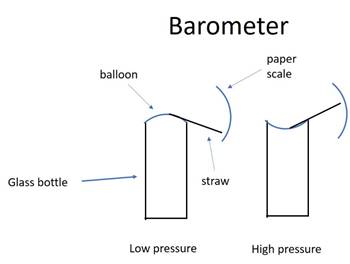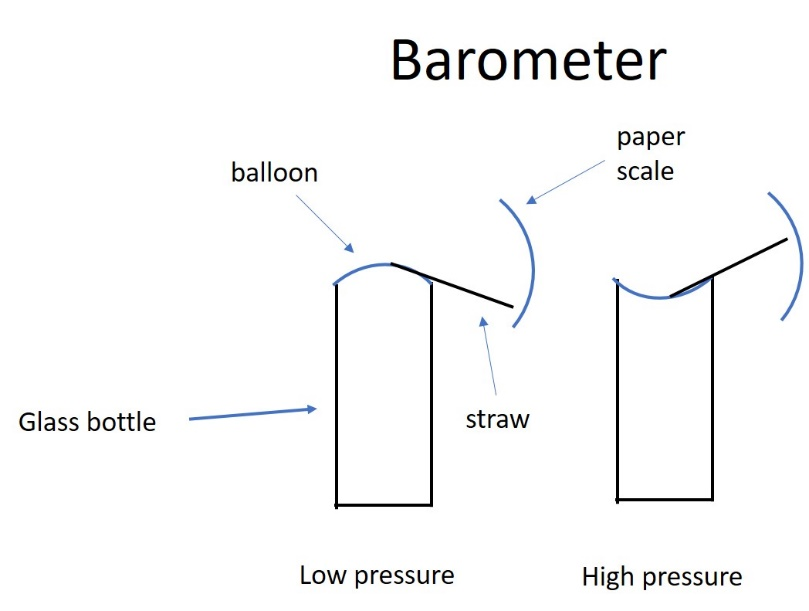# Input Quantities (Qi's) and Second-Order Perceptual Signals

[From Bruce Abbott (2017.02.27.1220 EST)]

As a kid in seventh grade my science fair project was a weather station, which included a barometer that I constructed from a milk bottle, a balloon, and a broom-straw as shown below:The balloon was stretched over the mouth of the bottle and the straw was then glued to the center of the balloon. A scale constructed from an index card was mounted behind the bottle to indicate the barometric pressure.

When the barometric pressure was low, the air pressure inside the bottle was greater than the pressure outside, causing the balloon to bulge outward. When the barometric pressure was high, the air pressure inside the bottle was lower than the pressure outside and the balloon was sucked inward. These changes caused the straw to change position as shown above.

The problem with this simple instrument is that the air pressure inside the bottle is not simply a function of the outside pressure – it also changes with temperature. If the temperature of the surrounding air is not constant, the position of the straw will depend on a combination of air temperature and barometric pressure. Something similar may happen with our sensory receptors. The problem for our perceptual systems then becomes how to extract a “pure” perceptual representation of a given variable from sensory inputs that are affected by more than one.

One way to resolve the problem occurs if we have another sensory input that only responds to one of the input variables. For example, assume that p for the first type of receptor is equal to the sum of variables X and Y. If the second receptor only responds to Y, then the perceptual system could derive a “pure” perception of X simply by subtracting the perception of Y from the perception of X+Y. In the case of my “bottle barometer,” this could be accomplished by getting a reading of the temperature of the air surrounding the bottle. We could then determine empirically how the instrument’s “perception” (straw position) depends on various combinations of air temperature and barometric pressure. We could then subtract the effect of the current air temperature to yield a pure measure of barometric pressure.

Bill Powers discussed a similar situation in Chapter 12 of Behavior: The Control of Perception. He imagines two first-order intensity signals, each of which is derived from a weighted combination of two input quantities, q1 and q2 using different weights:

s1 = a1q1 + b1q2

s2 = a2q1 + b2q2

Bill states the following:

Now let us sum the two signals, s1 and s2, in a second order input function, but using carefully chosen weights: let the first signal be weighted by a number proportional to b2 and the second be weighted negatively by a weight proportional to b2. The result will be a second-order signal S:

S = (a1b1 – a2b1)q1 [note that q2 does not appear]

Because of the special way the weights were chosen, the second order signal is a function of q1 only and is unaffected by variations of q2. In effect the second-order weighting can be adjusted to produce a signal analogous to the magnitude of a quantity once removed from the sensory interface. The first-order signals stand only for the magnitude of total stimulation from all sources at each input function; the second-order signal we have just created stands for quantities which are an immediate cause of the first-order input intensities. The fact that this can be done may mean that it is done by the nervous system.

With respect to my previous discussion of the input quantity, Qi, I think it worth noting that Bill is here using q1 and q2 to stand for the two environmental variables that together stimulate the sensory receptors and not the environmental analogs of the perceptual signals of the two types of receptor. Bill does not enforce a fixed interpretation of what the term “input quantity” means, using it in some cases to refer to the physical analog of the controlled perception (which may involve a combination of environmental variables) and in other cases using it to refer to the environmental variables themselves. This difference should cause no problem so long as one is clear what one means by these symbols in a given exposition.

Our perceptual systems perform highly sophisticated computations similar in principle (but much more complex) to those suggested above. These computations yield, for example, the perceptual constancies. In shape constancy, for instance, we perceive an object as retaining its essential shape even though its image on the retina is changing radically as we view the object from different angles.

Bruce